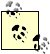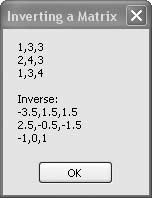# Recipe 6.32. Inverting a Matrix

#### Problem

You want to invert a matrix.

#### Solution

Sample code folder: Chapter 06\Matrix

Use the MatrixHelper. Inverse() function presented here and expanded upon in the MatrixHelper module presented in Recipe 6.35.

#### Discussion

The inverse of a matrix is another identically sized matrix that, when multiplied with the original matrix, gives the identity matrix. Only square matrices can be inverted. Matrix inversion is one of the basic matrix operations used for scientific, engineering, and computer graphics work. (A full description of matrices and their operations is beyond the scope of this book.)

Visual Basic 2005 is a good language for developing high-speed .NET Frameworkbased mathematical collections of number-crunching routines. It allows you to create fast-running classes, structures, and modules containing related functions or methods to meet many requirements. This recipe presents the code required to invert a matrix efficiently:

` Dim matrixA(,) As Double = { _    {1, 3, 3}, _    {2, 4, 3}, _    {1, 3, 4}} Dim matrixB(,) As Double = MatrixHelper.Inverse(matrixA) MsgBox(MatrixHelper.MakeDisplayable(matrixA) & _    vbNewLine & vbNewLine & "Inverse: " & _    vbNewLine & MatrixHelper.MakeDisplayable(matrixB)) `The MatrixHelper module is listed in its entirety in Recipe 6.35; it includes the Inverse() function and other functions called by Inverse().

Figure 6-32 shows the result of finding the inverse of a 3 x 3 matrix.

##### Figure 6-32. Finding the inverse of a square matrix with the MatrixHelper.Inverse( ) functionTo use the MatrixHelper.Inverse() function in your own applications, add the MatrixHelper module to your project and call the function from anywhere within your application.

See the full MatrixHelper.vb listing in Recipe 6.35.Visual Basic 2005 Cookbook: Solutions for VB 2005 Programmers (Cookbooks (OReilly))
ISBN: 0596101775
EAN: 2147483647
Year: 2006
Pages: 400

Similar book on Amazon